# Precalculus : Determine the Symmetry of an Equation

## Example Questions

### Example Question #1 : Determine The Symmetry Of An Equation

Is the following function symmetric across the y-axis? (Is it an even function?)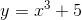This isn't even a function!

Cannot be determined from the information given

No

Yes

No

Explanation:

One way to determine algebraically if a function is an even function, or symmetric about the y-axis, is to substitute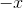in for. When we do this, if the function is equivalent to the original, then the function is an even function. If not, it is not an even function.

For our function: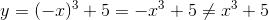Thus the function is not symmetric about the y-axis.

### Example Question #2 : Determine The Symmetry Of An Equation

Is the following function symmetric across the y-axis? (Is it an even function?)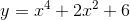No

There is not enough information to determine

That's not a function!

I don't know!

Yes

Yes

Explanation:

One way to determine algebraically if a function is an even function, or symmetric about the y-axis, is to substitutein for. When we do this, if the function is equivalent to the original, then the function is an even function. If not, it is not an even function.

For our function: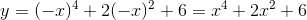Since this matches the original, our function is symmetric across the y-axis.

### Example Question #21 : Graphing Functions

Determine if there is symmetry with the equation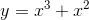to the-axis and the method used to determine the answer.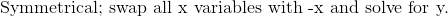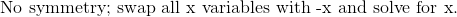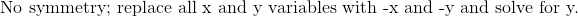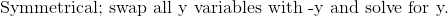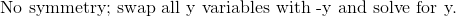Explanation:

In order to determine if there is symmetry about the x-axis, replace allvariables with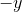.   Solving for, if the new equation is the same as the original equation, then there is symmetry with the x-axis.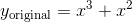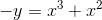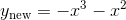Since the original and new equations are not equivalent, there is no symmetry with the x-axis.### Example Question #21 : Graphing Functions

Is the following function symmetrical about the y axis (is it an even function)?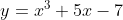Yes

No

Not a function

Insufficient Information

No

Explanation:

For a function to be even, it must satisfy the equality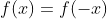Likewise if a function is even, it is symmetrical about the y-axis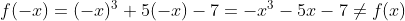Therefore, the function is not even, and so the answer is No

### Example Question #22 : Graphing Functions

Algebraically check for symmetry with respect to the x-axis, y axis, and the origin.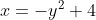No symmetry

Explanation:

For a function to be symmetrical about the y-axis, it must satisfy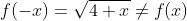so there is not symmetry about the y-axis

For a function to be symmetrical about the x-axis, it must satisfy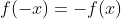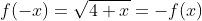so there is symmetry about the x-axis

For a function to be symmetrical about the origin, you must replace y with (-y) and x with (-x) and the resulting function must be equal to the original function.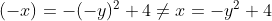### Example Question #31 : Graphing Functions

There is insufficient information to determine the answer

The given information does not include a function

Yes

No

### Example Question #32 : Graphing Functions

Which of the following best describes the symmetry ofwith respect to the x-axis, y axis, and the origin.

No symmetry

Explanation:

-For a function to be symmetrical about the y-axis, it must satisfy- For a function to be symmetrical about the x-axis, it must satisfy-For a function to be symmetrical about the origin, you must replace y with (-y) and x with (-x) and the resulting function must be equal to the original function.

### Example Question #33 : Graphing Functions

Symmetry about the x-axis and y-axis

Symmetry about the x-axis, y-axis, and origin

Symmetry about the y-axis and the origin

Explanation:

-For a function to be symmetrical about the y-axis, it must satisfy-For a function to be symmetrical about the x-axis, it must satisfy-For a function to be symmetrical about the origin, you must replace y with (-y) and x with (-x) and the resulting function must be equal to the original function.

-So there is no symmetry about the origin, and the credited answer is "symmetry about the y-axis"

### Example Question #1 : Determine The Symmetry Of An Equation

Algebraically check for symmetry with respect to the x-axis, y axis, and the origin.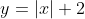Symmetry about the y-axis and origin

Symmetry about the x-axis, y-axis, and origin

Symmetry about the x-axis, and y-axis

Explanation:

For a function to be symmetrical about the y-axis, it must satisfy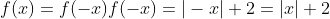so there is symmetry about the y-axis

For a function to be symmetrical about the x-axis, it must satisfy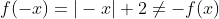so there is not symmetry about the x-axis

For a function to be symmetrical about the origin, you must replace y with (-y) and x with (-x) and the resulting function must be equal to the original function.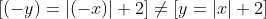So there is no symmetry about the origin.

### All Precalculus Resources